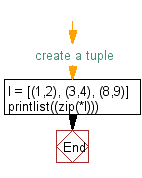﻿ Python: Unzip a list of tuples into individual lists - w3resource# Python Exercise: Unzip a list of tuples into individual lists

## Python tuple: Exercise-17 with Solution

Write a Python program to unzip a list of tuples into individual lists.

Sample Solution:-

Python Code:

``````#create a tuple
l = [(1,2), (3,4), (8,9)]
print(list(zip(*l)))
```
```

Sample Output:

```[(1, 3, 8), (2, 4, 9)]
```

Flowchart:Python Code Editor:

Have another way to solve this solution? Contribute your code (and comments) through Disqus.

What is the difficulty level of this exercise?

Test your Programming skills with w3resource's quiz.

﻿

## Python: Tips of the Day

Decapitalizes the first letter of a string:

Example:

```def tips_decapitalize(s, upper_rest=False):
return s[:1].lower() + (s[1:].upper() if upper_rest else s[1:])
print(tips_decapitalize('PythonTips'))
print(tips_decapitalize('PythonTips', True))
```

Output:

```pythonTips
pYTHONTIPS
```